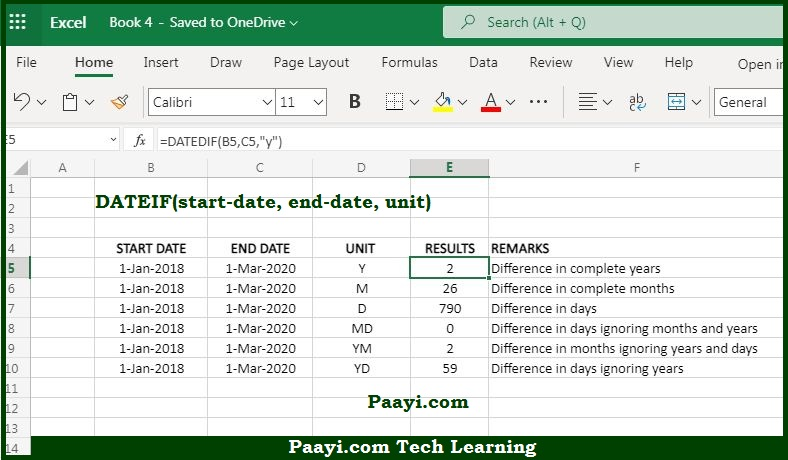# Learn How to Use Microsoft Excel DATEIF Function

Written by | 0 Comments | 753 Views

In this article, you will learn how to use the Microsoft Excel DATEIF function and its prime function in Microsoft Excel. You will also get to know the Microsoft Excel DATEIF function return value and syntax with the help of some examples.

## Microsoft Excel DATEIF Function

The prime use of the DATEIF function is to get days, months, or years between two dates. That means the Microsoft Excel DATEIF function returns the difference between two date values in years, months, and days. The DATEIF function is a compatibility function that comes from the Lotus1-2-3, and we don't know the reason when it is only documented in Excel 2000, and you can easily use it in all formulas in all the Excel versions.

### DATE Function Return Value

The return value is the number representing the time between the two dates.

### DATEIF Function Syntax

= DATEIF(start-date, end-date, unit)

Where:

• start-date: Start date in Excel date in number format
• end-date: End date in Excel date in number format.
• unit: The time unit that you can use - years, months, or days.

### How to Use Microsoft Excel DATEIF Function?

The DATEIF (Date + Diff) function is the compatibility function that works well with all the Excel versions. As per Chip Pearson, the DATEIF function is the drunk function in the Excel family, and Excel knows it is living happily but not spend time in polite conversation with it.

Before the move to the usages, let us understand the time units:

Time Units

The DATEIF function can calculate the time between the start date and the end-date in years, months and days. The time unit is specified in the unit argument and supplied as a text. The table below will make you understand the available unit values and the result for each. The time unit can be provided in upper or lower case:

Unit                             Result

"y"                               Difference in complete years

"m"                              Difference in complete months

"d"                               Difference in days

"MD"                           Difference in days ignoring months

"ym"                            Difference in moths, ignoring years

"yd"                             Difference in days, ignoring years

### Usage of DATEIF FunctionIn the example given above, column B contains the date January 1, 2018, and column C contains the date.